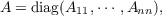#### Vol. 25, No. 2, 1968

 Download this articleFor screen For printingRecent Issues Vol. 307: 1  2 Vol. 306: 1  2 Vol. 305: 1  2 Vol. 304: 1  2 Vol. 303: 1  2 Vol. 302: 1  2 Vol. 301: 1  2 Vol. 300: 1  2Online Archive Volume: Issue:The Journal Editorial Board Subscriptions Officers Special Issues Submission Guidelines Submission Form Contacts ISSN: 1945-5844 (e-only) ISSN: 0030-8730 (print) Author Index To Appear Other MSP Journals
Invariance for linear systems of ordinary differential equations

### Al (Allen Frederick) Kelley, Jr.

Vol. 25 (1968), No. 2, 289–304
##### Abstract

In studying the existence and smoothness of invariant manifolds arising from nonlinear, perturbed systems of ordinary differential equations, one encounters the study of certain linear (in x), perturbation problems of the type

 𝜃 = a + 𝜖b(𝜃,𝜖) ẋ = (A + 𝜖B(𝜃,𝜖))x
where 𝜃 and x are vectors, A and B are matrices, b and B are multiply periodic in 𝜃, and 𝜖 is a perturbation parameter. Assuming A is a constant matrix consisting of square sub-matrices on the diagonal,with the maximum of the real parts of the eigenvalues of Ajj less than the minimum of the real parts of the eigenvalues of Akk for 1 j < k n; we construct a change of variables which reduces B to similar diagonal form.

Primary: 34.53
##### Milestones